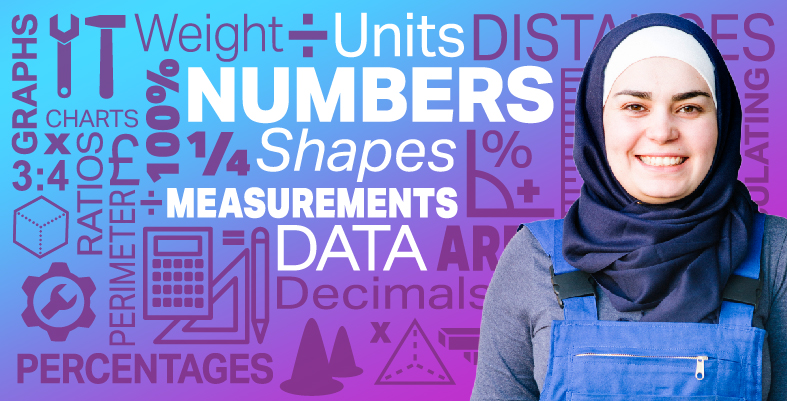Everyday maths for Construction and Engineering 1

Start this free course now. Just create an account and sign in. Enrol and complete the course for a free statement of participation or digital badge if available.

Free course

# 7 Averages

Sometimes it’s easier to present data numerically rather than graphically, and to find one number to represent a collection of data instead of lots of numbers. You can do this by finding the arithmetical average: ‘arithmetical’ means ‘doing sums’, and the ‘average’ is the representative value of all our data. So working out the arithmetical average means working out a representative value for your data with mathematical calculations.

Note: With data we talk about ‘data sets’, or sets of data. ‘Sets’ is just another word for ‘group’. So if we carried out a survey, we would have a data set.

You’ll be familiar with the word ‘average’. Outside maths, it is used to mean ‘not special’ or ‘just OK’. But in maths, ‘average’ means we can have one value that is representative of all our data and that uses all our data. You will also encounter the terms ‘mean average’, or just ‘mean’. The mean average is what we are referring to in this course and what we show you how to calculate below.

Where do we find averages in real life?

• If you look at a newspaper you will see that it will talk about the ‘average’ hours of sunshine in a day.
• A teacher might work out the average marks for students in a class.
• When you go on a journey you might talk about your average speed.
• The average goals scored per game over a season by your football team.

The arithmetic average is not difficult to work out. You need to do the following.

1. Add up all your data to a total. In the first example in the list above, about the number of hours of sunshine in a day, this could be the total number of hours of sunshine in August. (Let’s call this total ‘A’).
2. Add up the number of categories that your data falls into. Using the same example, this would be the number of days in August. (Let’s call this amount ‘B’).
3. Divide the total of your data (A) by the number of bits of data (B). So A ÷ B = the average. In the example about the average hours of sunshine in August, if there were 434 total hours of sunshine, divided by 31 (the number of days in August), there would be an average of 14 hours of sunshine in a day in August.

Have a look at the example below, where you will be looking at the average hours of sunshine.

## Example: Average hours of sunshine

The hours of sunshine per day during one week’s work on site in Torquay in June was recorded as follows:

DayHours of sunshine
Sunday6
Monday1
Tuesday7
Wednesday8
Thursday5
Friday2
Saturday6

You could draw a bar chart or a line graph to present this data. However – as you might expect from the British weather – the amount of sunshine varied a lot from day to day.

It might be more useful to find out the average amount of sunshine per day. This would give you one value, which you could use as a guide as to how much sunshine to expect per day.

### Method

To work out this average value you need to:

• add up the amount of sunshine for each day
• divide this by the number of days you have the data for.

With this example we have:

• 6 + 1 + 7 + 8 + 5 + 2 + 6 = 35 hours of sunshine for the week

and seven days of data. So, the average is:

• 35 ÷ 7 = 5 hours

Note: You must remember what units you are working in and write in these units after your average value – otherwise, it won’t make sense.

So from this data you can see that, on average, there were five hours of sunshine per day in a week in June in Torquay. You could then use that information to help choose the best schedule for a site. If you needed more than five hours of sunshine to be productive, you may decide to select a better month in which to schedule the work.

### Method summary

• Find out the number of categories that your data falls into.
• Divide the total of your data by the number of categories of data to give the average.
• Don’t forget to put what units you are working in, for example hours, goals, people, etc.
FSM_SSC_1